##Special case of Desargues involution theorem

Actually this is a special case of the special case discussed in DesarguesInvolution2.html . There we saw that a line (e) intersects the conics passing through {A,B,C} and tangent to a given line (t) at A, in pairs of points in involution.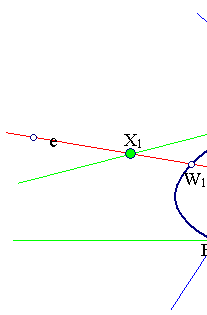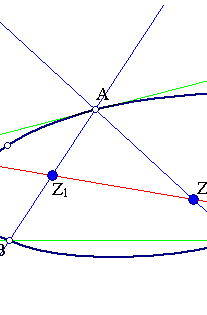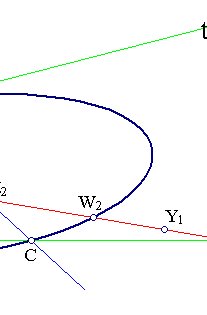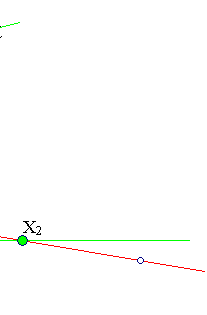Letting C coincide with B we obtain the figure below, in which Z1, Z2 coincide with Z.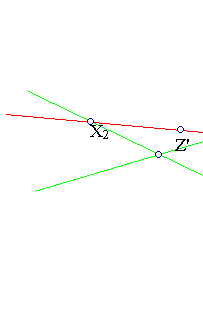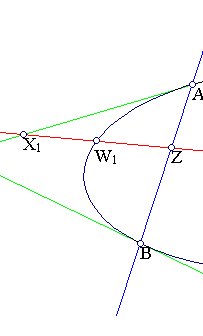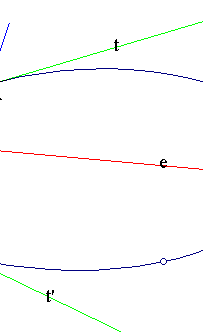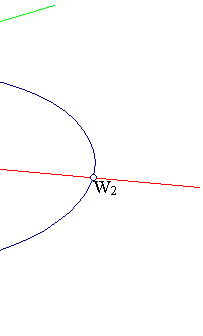Desargues theorem now asserts that Z is a double point of the involution defined on line (e) by the intersection points W1, W2 of conics passing through {A,B} and being there tangent to two given lines.
Note that the other fixed point Z' of the involution on (e) is the harmonic conjugate of Z with respect to W1, W2 (or X1, X2). This can be seen by considering the circle bundle generated by the two circles with diameters W1W2 and X1X2. The reason for this is explained in InvolutionBasic2.html .
The above property can be used to construct the conic passing through three points and two tangents. This is discussed in Conic3Pts2Tangents.html

### See Also

Conic3Pts2Tangents.html
CrossRatio0.html
DesarguesInvolution.html
DesarguesInvolution2.html
FourPtsAndTangent.html
FourPtsAndTangent2.html
HomographicRelation.html
HomographicRelationExample.html
InvolutionBasic.html
InvolutionBasic2.html

### References

Doerrie, Heinrich 100 Great Problems of Elementary Mathematics New York, Dover, 1965, p. 269.

 Produced with EucliDraw© http://users.math.uoc.gr/~pamfilos/